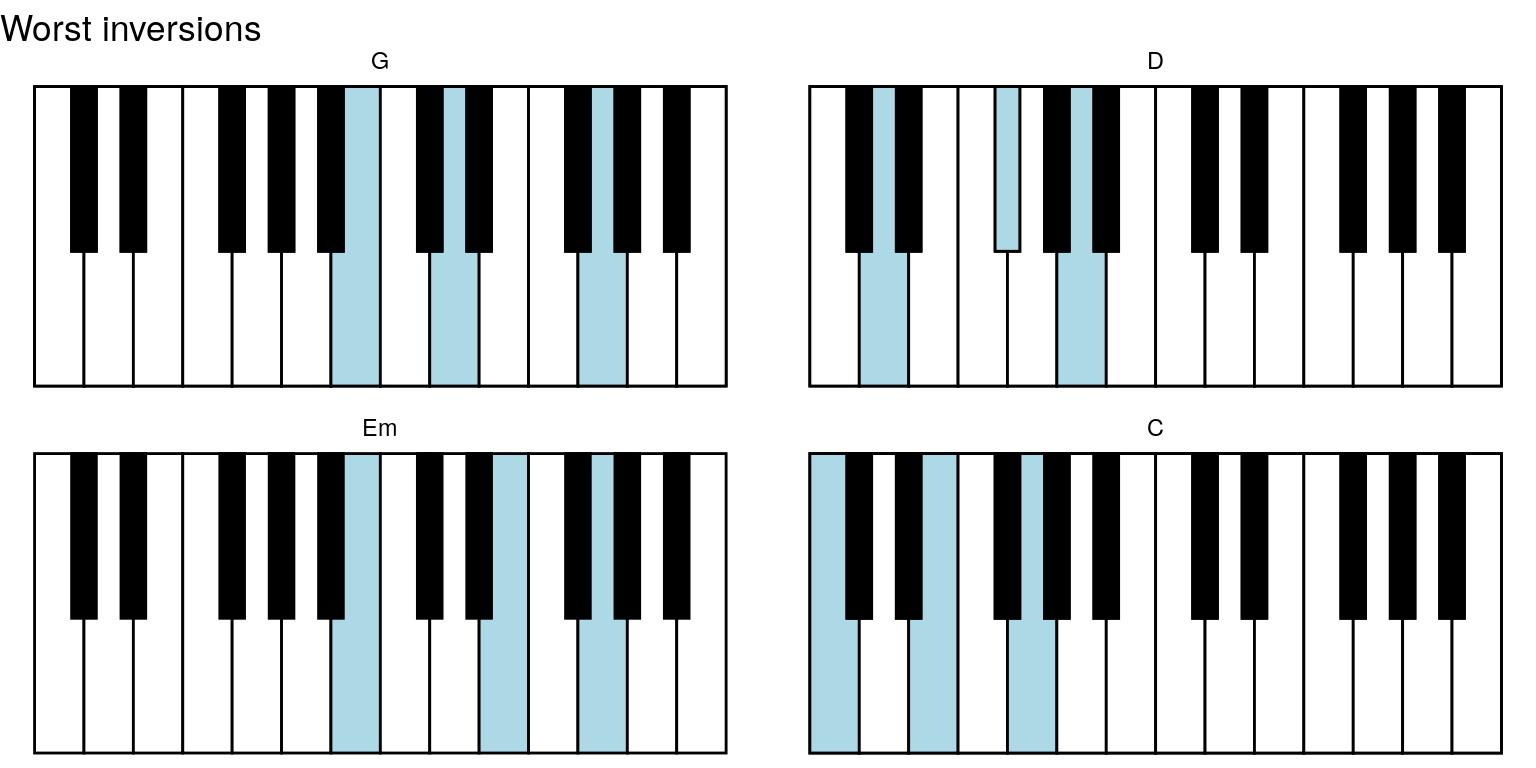Want to share your content on R-bloggers? click here if you have a blog, or here if you don't.

I am learning how to play piano.
In that process, I needed charts with piano chords. I know it is good practise to construct the chords manually, figure out the inversions and so on, but I found that some aspects of learning were improved with a chord chart.
I searched the (entire!) internet, and struggled to find good, customisable charts.

Thus I had to make something myself: `pichor`, an `R` package for making and working with piano chords in `R`.

Please find the package’s website at https://mikldk.github.io/pichor (with various tutorials/vignettes at https://mikldk.github.io/pichor/articles).
The source code can be found at https://github.com/mikldk/pichor.
Install the package with the `R` command `devtools::install_github('mikldk/pichor')` (when `devtools` package is installed).

Below, I will briefly demonstrate a few examples.

## Examples

### Piano chart

`library(pichor)`

There is built-in data in `keys_chords` with information about some of the keys on a piano.
These can be used for e.g. drawing a diagram of a piano:

`ggpiano(keys_chords)`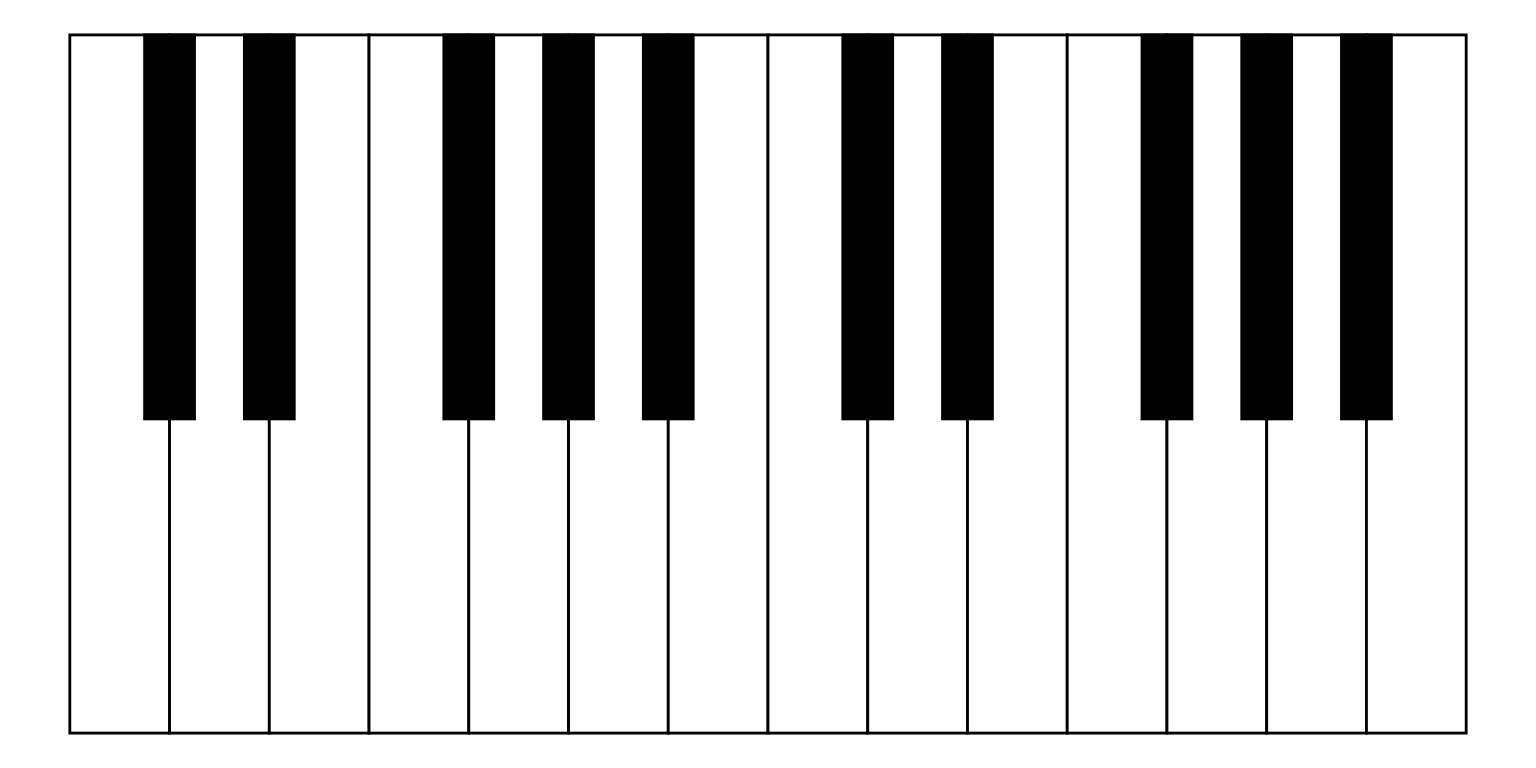It is also possible to highlight certain keys (and show labels):

```keys_chords_highlighted <- highlight_keys(keys_chords, keys = c(7, 10, 14))
ggpiano(keys_chords_highlighted, labels = TRUE)```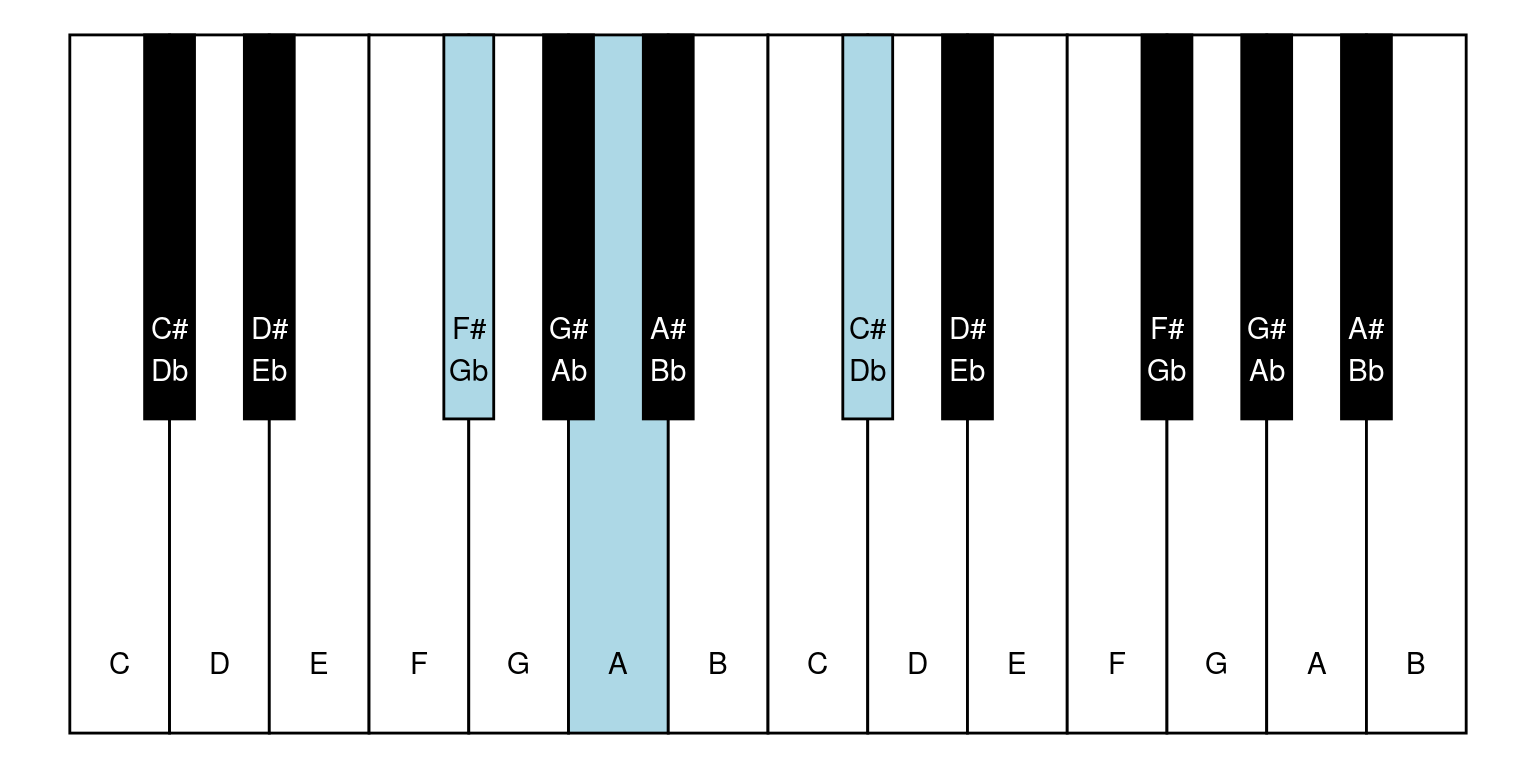The above is also possible using `magrittr`’s pipe, `%>%`:

```library(magrittr)

keys_chords %>%
highlight_keys(keys = c(7, 10, 14)) %>%
ggpiano(labels = TRUE)```### Working with chords

It is possible to work with chords, e.g. getting keys, the highest tone etc.:

```chrd <- construct_chord_raw(root_tone = "F#", distances_rel = c(4, 3))
chrd
## F# chord with tones F#/Gb, A#/Bb, C#/Db
as.character(chrd, brief = TRUE)
##  "F#"
chrd_Fsm <- construct_chord_minor(root_tone = "F#")
chrd_Fsm
## F#m chord (minor) with tones F#/Gb, A, C#/Db
as.character(chrd_Fsm)
##  "F#m chord (minor) with tones F#/Gb, A, C#/Db"
as.character(chrd_Fsm, brief = TRUE)
##  "F#m"
get_keys(chord = chrd_Fsm)
##   7 10 14
get_keys_highest_tone(chord = chrd_Fsm, highest_tone = "A")
##   2  7 10```

And instead of highlight keys, chords can be highlighted, too:

```keys_coords %>%
highlight_chord(chord = chrd_Fsm) %>%  # root form by default
ggpiano()```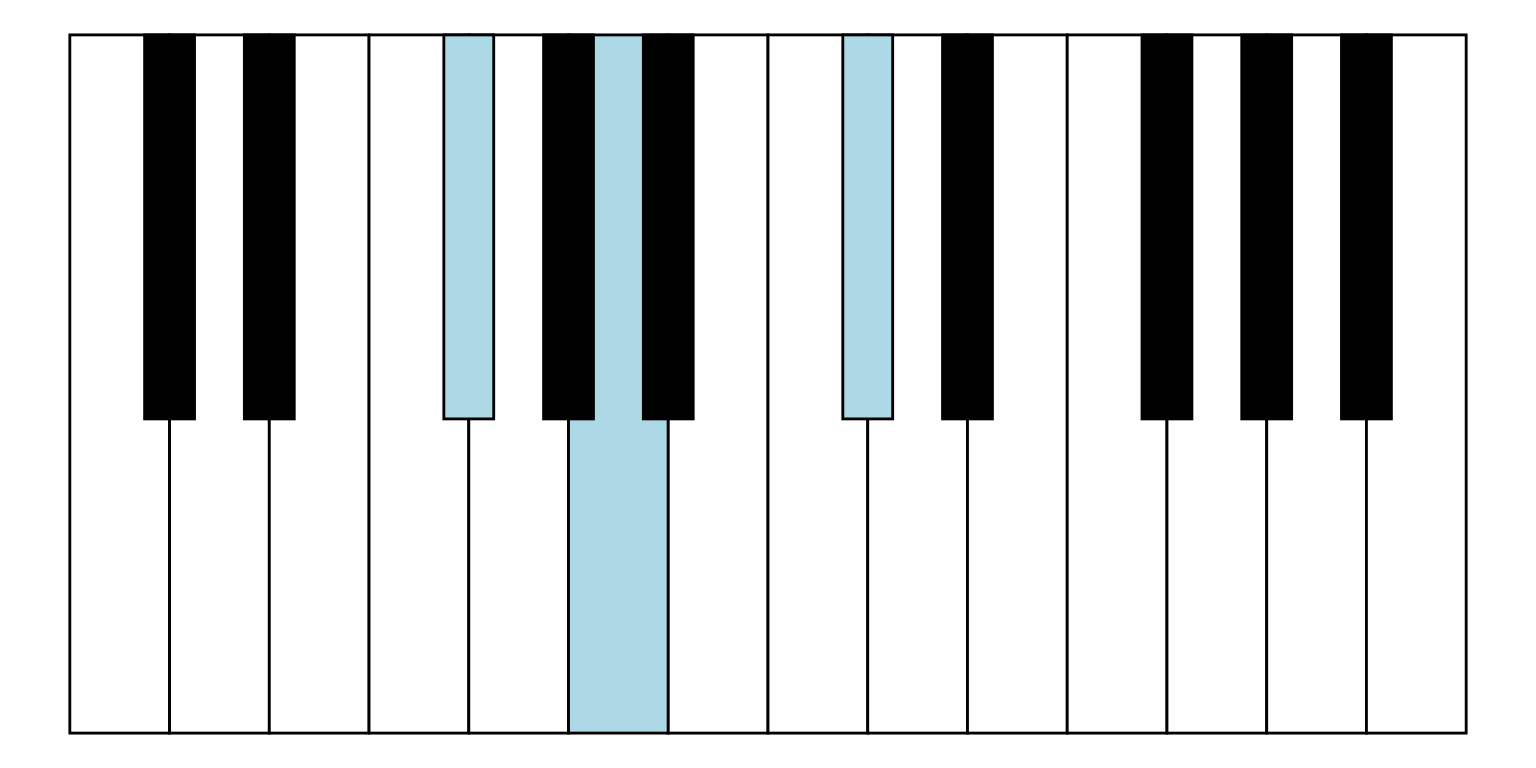```keys_coords %>%
highlight_chord(chord = chrd_Fsm, highest_tone = "A") %>%
ggpiano()```It is also possible to work with chord inversions:

```chrd_Bm <- construct_chord_minor(root_tone = "B")
get_keys(chord = chrd_Bm)
##  12 15 19
get_keys_inversion(chord = chrd_Bm, inversion = 0)
##  12 15 19
get_keys_inversion(chord = chrd_Bm, inversion = 1)
##   3  7 12
get_keys_inversion(chord = chrd_Bm, inversion = 2)
##   7 12 15```

These can also be highlighted in a chart:

```keys_coords %>%
highlight_chord(chord = chrd_Bm, inversion = 2L) %>%
ggpiano()```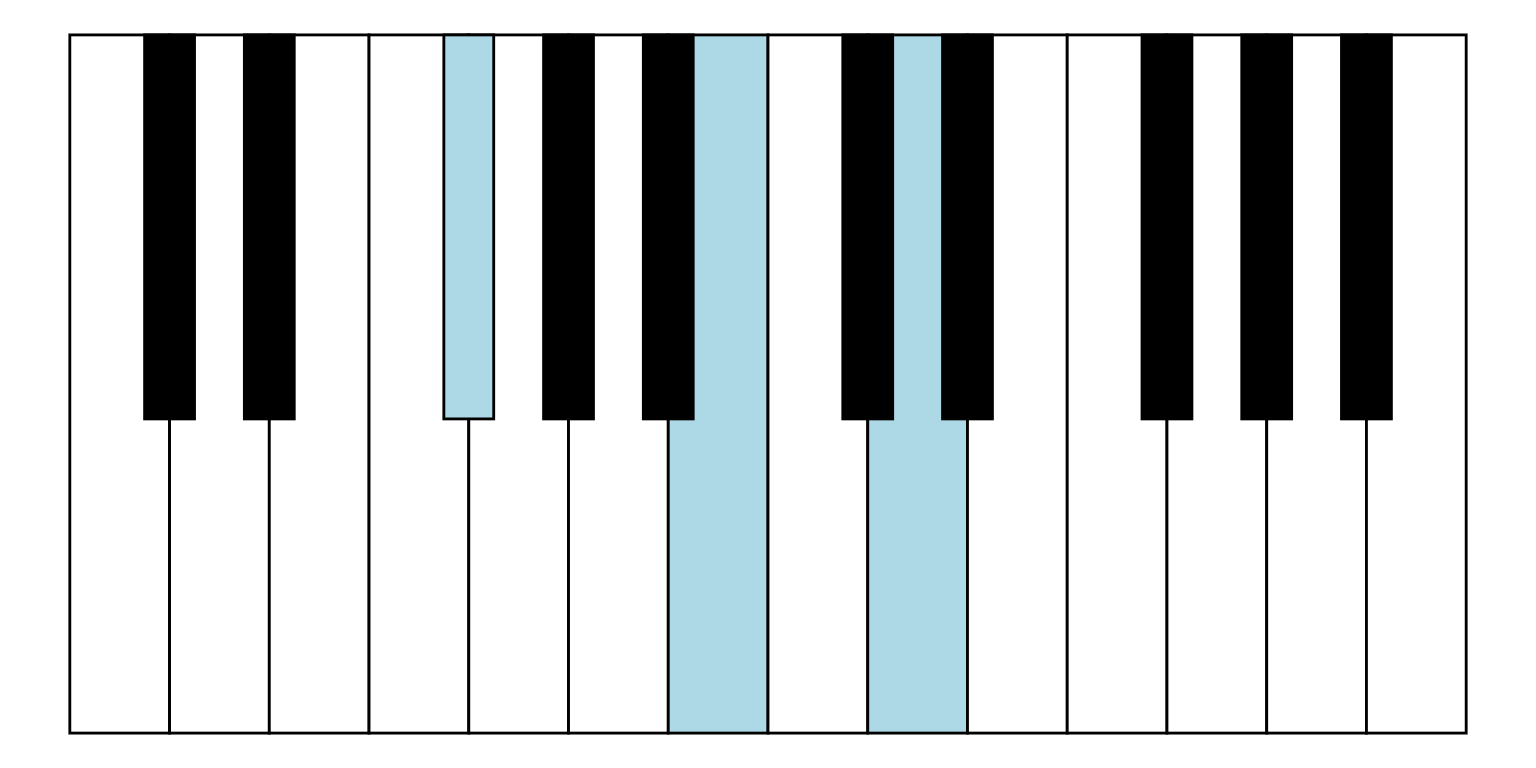### Chord sequence

It is also possible to work with a sequence of chords.
Let me illustrate it with a version of “Let it be” by Beatles that initially has chords G, D, Em, C, G, D, C.

```chords <- list(construct_chord_major("G"),
construct_chord_major("D"),
construct_chord_minor("E"), # Em
construct_chord_major("C"),
construct_chord_major("G"),
construct_chord_major("D"),
construct_chord_major("C"))
chords
## []
## G chord (major) with tones G, B, D
##
## []
## D chord (major) with tones D, F#/Gb, A
##
## []
## Em chord (minor) with tones E, G, B
##
## []
## C chord (major) with tones C, E, G
##
## []
## G chord (major) with tones G, B, D
##
## []
## D chord (major) with tones D, F#/Gb, A
##
## []
## C chord (major) with tones C, E, G
chord_names <- sapply(chords, as.character, brief = TRUE)```

`key_seq <- lapply(chords, get_keys)`

For more advanced plotting and data wrangling, `ggplot2` and `dplyr` are first loaded.
Helpful facet panel titles are providing by `sequence_names` argument to `highlight_key_sequence()`:

```library(ggplot2)
library(dplyr)

keys_chords %>%
highlight_key_sequence(key_sequence = key_seq,
sequence_names = chord_names) %>%
ggpiano() +
facet_wrap(~ seq_name)
## NULL```And for example include `seq_no` in a new column `seq_lbl` (that are then converted to a factor with the right ordering):

```keys_chords %>%
highlight_key_sequence(key_sequence = key_seq,
sequence_names = chord_names) %>%
mutate(seq_lbl = paste0(seq_name, " (#", seq_no, ")")) %>%
mutate(seq_lbl = forcats::fct_inorder(seq_lbl)) %>%
ggpiano() +
facet_wrap(~ seq_lbl)
## NULL```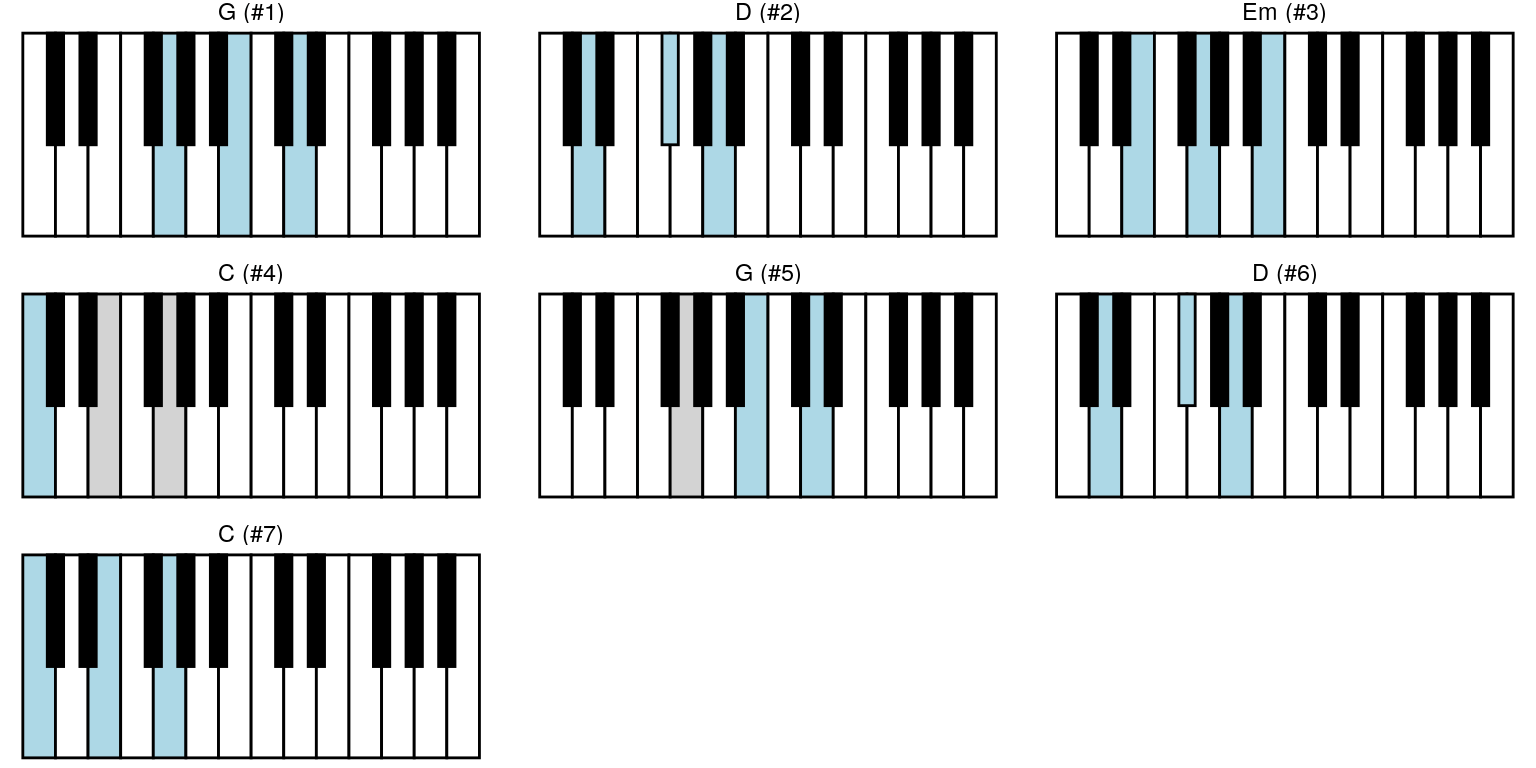#### Finding better inversions

Currently only one method is available for finding better inversions.
It is minimising the distances between keys in consecutive chords, where
distance is the number of keys not used anymore plus new keys.
And with an exhaustive approach that is only feasible for shorter sequences.

`opt_res <- optim_min_dist_exhaustive(key_sequence = key_seq)`

```keys_chords %>%
highlight_key_sequence(key_sequence = opt_res\$best,
sequence_names = chord_names) %>%
ggpiano() +
facet_wrap(~ seq_name) +
labs(title = "Best inversions")
## NULL``````keys_chords %>%
highlight_key_sequence(key_sequence = opt_res\$worst,
sequence_names = chord_names) %>%
ggpiano() +
facet_wrap(~ seq_name) +
labs(title = "Worst inversions")
## NULL```